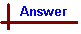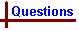For on-line help with this topic, see the chemcal module "Stoichiometry" which deals with moles; balancing equations; stoichiometric calculations; molarity and solution stoichiometry.   MOLE CONCEPT (Advanced Questions 1) Your feedback on these self-help problems is appreciated. Click here to send an e-mail. Shortcut to Questions Q: 1 2 3 4 5 6 7 8 9 10 11 1 (1.) What mass of methanol (CH3OH) must be added to 2.5 mole of ethanol (C2H5OH) in order to produce a solution containing equal numbers of molecules of the two species? (2.) Assuming no chemical reaction occurs, what is the total number of molecules present in the resulting solution?2 The diatomic molecule of an element Z has a mass of 6.31 x 10-23g. What is the atomic weight of the element Z?3 Hydrogen chloride gas (7.3 g) is in a container at a pressure of 2.5 atmosphere and a temperature of 300 K. (1.) How many moles of gas are present? (2.) How many molecules of gas are present? (3.) If all the gas were dissolved in 100 cm3 of water, what mass of silver nitrate would need to be added to precipitate all the chloride ion produced.4 What is the mass in g of each of the following at 273 K and 101 kPa? (1.) neon (22.4 litre). (2.) ice (22.4 litre). (3.) mercury (0.10 mol).5 Formic acid (HCOOH) is a liquid monoprotic acid. (1.) What amount (in mole) of formic acid is present in 1.00 litre of the pure substance at 298 K? (2.) What mass of formic acid should be taken and diluted to obtain 1.00 litre of a 0.075 M solution?6 How many atoms are in the following at 273 K and 101 kPa? (1.) neon (40.4 g) (2.) silver (54 g) (3.) fluorine (22.4 litre) (4.) iodine (127 g)7 Hydrogen iodide is a gas at room conditions. If 5.0 litre of hydrogen iodide at 298 K and 760 mmHg is dissolved in water and the volume made up to 1.000 litre, what is the molarity of the solution?8 A certain hydrate of sodium carbonate (2.433 g) was dried in an oven at 383 K. The resulting anhydrous sodium carbonate was found to have a constant weight of 1.112 g. What is the formula of the hydrated salt?9 Magnesium oxide (2.00 g) is added to 10.0 cm3 of 2.00 M hydrochloric acid. Calculate which reactant is in excess and the number of mole by which it is in excess.10 Pure formic acid (HCOOH), is a liquid monoprotic acid decomposed by heat to carbon dioxide and hydrogen. (1.) What mass of the pure acid should be diluted with water to produce 1.00 litre of 2.00 M aqueous solution of formic acid? (2.) What volume of 0.250 M sodium hydroxide would be required to react exactly with 30.0 cm3 of this dilute solution of formic acid? (3.) What is the maximum volume of carbon dioxide at 273 K and 1.00 atmosphere that could be obtained by heating 1.00 mole of formic acid? (4.) How many molecules of carbon dioxide would it contain?11 Barium carbonate (5.50 g) is added to 100 cm3 of 0.60 M nitric acid. Calculate which reactant is in excess and the number of mole by which it is in excess.MOLE CONCEPT (Advanced Answers 1) 1 In order to produce a solution containing equal numbers of molecules, there needs to be the same number of moles of each species, therefore 2.5 moles methanol will need to be added to the ethanol. Mass CH3OH required = moles CH3OH x molar mass CH3OH = 2.5 mol x 32.04 g mol-1 = 80 g. No. molecules in the solution = no. moles of all species x NA = (moles CH3CH2OH + moles CH3OH) x NA = (2.5 mol + 2.5 mol) x 6.022 x 1023 = 3.0 x 1024 molecules.2 Since the element is in diatomic form, Z2, each atom will weigh half the molecular mass of the diatomic molecule, ie mass of 1 atom of Z = 0.5 x 6.31 x 10-23 g = 3.16 x 10-23 g. The gram atomic mass (molar mass) of the element is the mass of one mole of Z atoms = mass of elemental atom x NA = 3.16 x 10-23 x 6.022 x 1023 = 19.0 g mol-1 and the atomic weight of Z = 19.0.3 (1.) Moles HCl = mass HCl / molar mass HCl = 7.3 g / 36.46 g mol-1 = 0.20 mol. (2.) No. molecules HCl = moles HCl x NA = 0.20 mol x 6.022 x 1023 = 1.20 x 1023 mlecules . (3.) Balanced equation for the reaction: AgNO3 + HCl → AgCl + HNO3 Silver chloride is insoluble and precipitates as a solid. From the above equation, 1 mol HCl reacts with 1 mol AgNO3, therefore moles AgNO3 required = moles HCl = 0.20 mol. Mass AgNO3 required = moles AgNO3 x molar mass AgNO3 = 0.20 mol x 169.91 g mol-1 = 34 g.4 (1.) Using the ideal gas approximation: moles Ne (273 K, 101 kPa) = volume Ne / molar volume = 22.4 L / 22.4 L mol-1 = 1.00 mol. Mass Ne = moles Ne x molar mass Ne = 1.00 mol x 20.18 g mol-1 = 20.2 g. (2.) Note that ice is a solid, not a gas - do not be mislead into using the molar volume of an ideal gas which is 22.4 L at these conditions. While the molar volume of all ideal gases is independent of the gas used, this is not true of liquids or solids. Instead, the density of each particular solid or liquid substance must be available in order to calculate any mass - volume relationship. Using the density of ice at 273 K: Mass H2O (at 273 K) = volume ice x density ice = 22,400 cm3 x 0.917 g cm-3 = 2.05 x 104 g. (3.) Mass Hg = moles Hg x molar mass Hg = 0.10 x 200.6 = 20 g.5 (1.) Mass HCOOH (298 K) = density HCOOH x volume HCOOH = 1.220 g cm-3 x 1000 mL = 1.22 kg (note that 1 cm3 = 1 mL, 1000 cm3 = 1 L). Moles HCOOH = mass HCOOH / molar mass HCOOH = 1.22 kg / 46.03 g mol-1 = 26.5 mol. (2.) 0.075 M means 0.075 moles of solute per litre of solution. Therefore 0.075 mol is HCOOH required. Mass HCOOH = moles HCOOH x molar mass HCOOH = 0.075 mol x 46.03 g mol-1 = 3.5 g.6 (1.) Moles Ne = mass Ne / molar mass Ne = 40.4 g / 20.18 g mol-1 = 2.00 mol. No. atoms Ne = moles Ne x NA = 2.00 mol x 6.022 x 1023 = 1.20 x 1024 atoms. (2.) Moles Ag = mass Ag / molar mass Ag = 54 g / 107.9 g mol-1 = 0.50 mol. No. atoms Ag = moles Ag x NA = 0.50 mol x 6.022 x 1023 = 3.0 x 1023 atoms. (3.) Note that fluorine exists as diatomic F2 (not F) in it's ordinary gaseous state. Moles F2 (273 K, 101 kPa) = volume F2 / molar volume = 22.4 L / 22.4 L mol-1 = 1.00 mol. No. molecules F2 = moles F2 x NA = 1.00 mol x 6.022 x 1023 = 6.02 x 1023 atoms. No. atoms F = no. molecules F2 x 2 = 6.02 x 1023 x 2 = 1.20 x 1024 atoms. (4.) Iodine is a diatomic solid, I2, Moles I2 = mass I2 / molar mass I2 = 127 g / (126.9 X 2)g mol-1 = 0.500 mol. As iodine is diatomic, no. of I atoms = 2 x moles I2 x NA = 2 x 0.500 x 6.022 x 1023 = 6.02 x 1023 atoms.7 Using the ideal gas approximation: moles HI (298 K, 760 mmHg) = volume HI / molar volume = 5.0 L / 24.5 L mol-1 = 0.20 mol. Therefore there will be 0.20 moles HI dissolved in 1.000 L H2O, so the solution will be 0.20 M.8 The formula of anhydrous sodium carbonate is Na2CO3. The difference in mass between the original hydrated salt, and the dry anhydrous salt is due solely to lost water. Mass of H2O lost = mass hydrated salt - mass anhydrous salt = 2.433 g - 1.112 g = 1.321 g. Moles H2O lost = mass H2O / molar mass H2O = 1.321 g / 18.02 g mol-1 = 0.07331 mol. Moles Na2CO3 present in dried sample = mass Na2CO3 / molar mass Na2CO3 = 1.112 g / 105.99 g mol-1 = 0.01049 mol. The ratio of H2O to Na2CO3 = moles H2O / moles Na2CO3 = 0.07331 / 0.01049 = 6.989 / 1 Taking into consideration the error implicit in the given masses, as well as the possibility of rounding errors, this figure which must be an integer is taken as 7, hence providing the hydrated salt formula Na2CO3.7H2O.9 The oxides of metals react with acids to form a salt and water. The stoichiometric ratios of the reactants is found from the balanced equation: MgO + 2HCl → MgCl2 + H2O Moles MgO = mass MgO / molar mass MgO = 2.00 g / 40.31 g mol-1 = 0.0496 mol. Moles HCl = molarity HCl x volume HCl solution (L) = 2.00 M x ((10.0 cm3) / 1000 cm3) = 0.0200 mol. From the equation, 1 mol MgO reacts with 2 mol HCl, therefore 0.0496 mol MgO requires 2 x 0.0496 = 0.0992 mol HCl for complete reaction. Since only 0.0200 mol HCl is present, MgO is present in excess.10 (1.) 2.00 M means there are 2.00 moles HCOOH dissolved per litre of solution. Mass HCOOH = moles HCOOH x molar mass HCOOH = 2.00 mol x 46.03 g mol-1 = 92.1 g. (2.) Balanced equation: HCOOH + NaOH → HCOONa + H2O From this equation, 1 mol NaOH will react with 1 mol HCOOH. Moles HCOOH = volume solution x molarity = 0.0300 L x 2.00 M = 0.0600 mol. Therefore 0.0600 mol NaOH is required for reaction. Volume of 0.250 M NaOH solution required = moles NaOH required / molarity of NaOH solution = 0.0600 mol / 0.250 mol L-1 = 0.240 L. (3.) Balanced equation for thermal decomposition: HCOOH → H2 + CO2 From the equation, 1 mol HCOOH produces 1 mol gaseous CO2. From the ideal gas approximation, 1 mol gas occupies 22.4 L at 273 K, 1.00 atm. (4.) 1 mol CO2 contains NA molecules = 6.022 x 1023 molecules.11 The stoichiometric ratios of the reactants is found from the balanced equation: BaCO3 + 2HNO3 → Ba(NO3)2 + H2O + CO2 Moles BaCO3 = mass BaCO3 / molar mass BaCO3 = 5.50 g / 197.31 g mol-1 = 0.0279 mol. Moles HNO3 = molarity HNO3 x volume HNO3 solution (L) = 0.60 M x ((100 cm3) / 1000 cm3) = 0.060 mol. From the equation, 1 mol BaCO3 reacts with 2 mol HNO3, therefore 0.0279 mol BaCO3 requires 2 x 0.0279 = 0.0558 mol HNO3 for complete reaction. There is 0.060 mol HNO3 present, so HNO3 is in excess by (0.060 - 0.0558) = 0.004 mol.